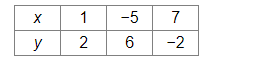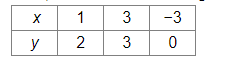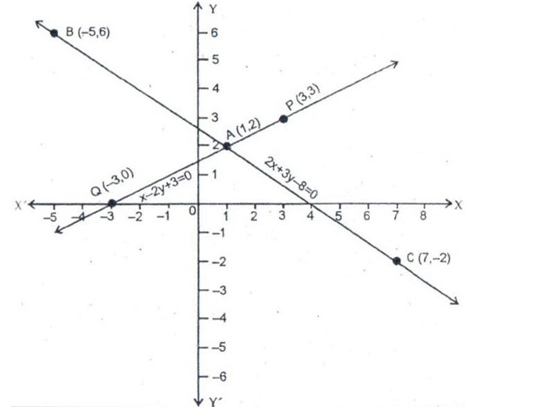# Solve this

Question:

$2 x+3 y=8$

$x-2 y+3=0$

Solution:

On a graph paper, draw a horizontal line X'OX and a vertical line YOY' as the x-axis and y-axis, respectively.

Graph of $2 x+3 y=8$

2x + 3y = 8
⇒ 3y = (8 − 2x)

$\therefore y=\frac{8-2 x}{3}$    .............(i)

Putting x = 1, we get y = 2.
Putting x = −5, we get y = 6.
Putting x = 7, we get y = −2.
Thus, we have the following table for the equation 2x + 3y = 8.Now, plot the points A(1, 2), B(5, −6) and C(7, −2) on the graph paper.
Join AB and AC to get the graph line BC. Extend it on both ways.
Thus, BC is the graph of 2x + 3y = 8.

Graph of $x-2 y+3=0$

− 2y + 3 = 0
⇒ 2y = (x + 3)

$\therefore y=\frac{x+3}{2}$   ..........(ii)

Putting x = 1, we get y = 2.
Putting x = 3, we get y = 3.
Putting x = −3, we get y = 0.
Thus, we have the following table for the equation − 2y + 3 = 0.Now, plot the points P(3, 3) and Q(−3, 0). The point A(1, 2) has already been plotted. Join AP and QA and extend it on both ways.
Thus, PQ is the graph of − 2y + 3 = 0.The two graph lines intersect at A(1, 2).
∴ x = 1 and y = 2